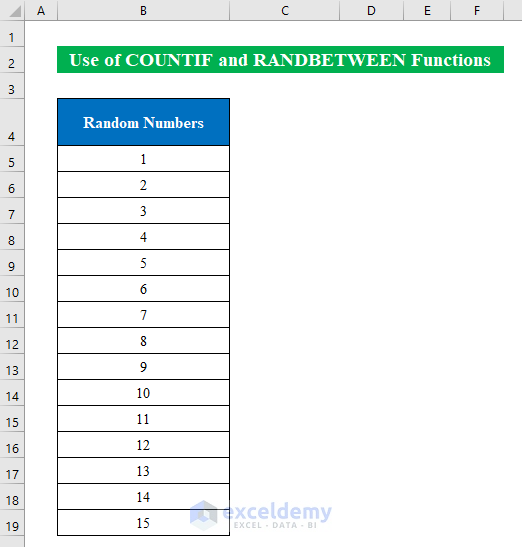# Probability Formula for Lottery in Excel (3 Instances)

While working in Microsoft Excel, we sometimes need to calculate the probability of a lottery with simple formulas. But it might seem difficult to determine the actual probability. Today, in this article, I am sharing with you how to calculate the probability of lottery with formula in Excel.

## 3 Ways to Use Probability Formula for Lottery in Excel

In the following, I have described 3 simple and easy methods to calculate the probability with formula for lottery in Excel.

Suppose we have a Range in our table “Range 1 to” and “# to Win”. Now using the formula we will calculate the total combination between numbers.### 1. COMBIN Function to Calculate Probability with a Combination of Lottery

If you are looking for a simple solution to calculate the probability then you can use the COMBIN function. The COMBIN function will find the total possible number of groups from the given values. Follow the instructions below-

Steps:

• First, select a cell (D5) and apply the following formula-
`=COMBIN(B5:C5)`• Second, hit ENTER and drag the “Fill Handle” down.
• Therefore, we have the total combination for the given numbers.Here we found the total combinations between these two numbers without any repetitions. Thus the probability between these numbers can be found.

### 2. Combination of RAND, RANK, COUNTIF and VLOOKUP Functions for Lottery Probability Formula

We often look for solutions to find lottery numbers. In order to do so combine RAND, RANK, COUNTIF, and VLOOKUP functions to determine the probability of winning the lottery.

Suppose we have a table where we will generate “Random Numbers” and “Unique Rank”. Hence, determining the “Ball Number” will lead us to calculate the probability of winning the lottery.Step 1:

• Presently, select a cell (B5) and write the below formula to generate random numbers-
`=RAND()`• In the same fashion, click ENTER and pull the “Fill Handle” down.
• Within a moment you will find the random numbers in the selected column.• Thereafter, we will determine the unique rand by putting the following formula inside the cell (C5)-
`=RANK(\$B5,\$B\$5:\$B\$17)+COUNTIF(C\$4:C4,C4)-1`

Where,

• The COUNTIF function will count cells for the given condition- COUNTIF(C\$4:C4,C4)-1 and return an output 0.
• The RANK function will return the position from the argument- RANK(\$B5,\$B\$5:\$B\$17) and provide a unique argument from cells (B5:B17).• Gently, press ENTER and drag the “Fill Handle” below to fill all the cells.
• Thus, we will find the proper unique rand from the random numbers.Step 2:

• In the screenshot below we just filled the “Ball Number” sequentially.• In this final step, let’s find the lottery numbers utilizing the VLOOKUP function.
• For that, choose a cell (G5) and put the formula down-
`=VLOOKUP(G5,\$C\$4:\$D\$17,2,FALSE)`

Where,

• The VLOOKUP function will search for a given value from the leftmost column (C) and return a value in the same row.• Hence, click ENTER and drag the “Fill Handle” horizontally.
• In summary, we will get the lottery number in the chosen column. Simple isn’t it?### 3. Apply COUNTIF and RANDBETWEEN to Generate and Calculate Probability of Lottery

Lottery prediction algorithms won’t guarantee a win all the time but you can determine the probability more accurately by utilizing the COUNTIF and RANDBETWEEN functions.

Imagine a table with random numbers sequentially. Now we will generate some random numbers and search for the probability within those numbers.

###Step 1:

• To start with, select a cell (B21) and apply the formula down-
`=RANDBETWEEN(1,15)`• Simply, click ENTER and drag the “Fill Handle” row and column wise to fill.
• Now, selecting those newly created random numbers click CTRL+C to copy.• Then, press the right button of the mouse and choose “Values” to paste as values. Thus, the random numbers won’t change automatically.Step 2:

• Let’s determine the instances by applying the below formula in a new cell (C5)-
`=COUNTIF(\$B\$21:\$G\$30,B5)`• Hence, hit ENTER and drag down the “Fill Handle” to fill the column.• After getting the count of cells we will apply conditional formatting to get the highest numbers for instances.
• Choose cells (C5:C19) and select “Above Average” from the “Conditional Formatting” rules.• In this way, you will get the highest random numbers counted in the cell. Now choosing those numbers we will repeat the process again to find a better probability.
• Thus choose the cells and copy them in a new column following the screenshot.• Finally, you will get more filtered numbers from the generated numbers.
• To finish, we will check the probability with the simple formula below-
`=F8/COUNT(B21:G30)`

Where,

• The COUNT function will count the number of cells and divide it with the random numbers to find the actual probability.• Simply hit ENTER.
• In conclusion, we have the numbers and the probability for the lottery in our excel worksheet.## Things to Remember

• To prevent the lottery numbers from changing you can change the “Calculation Options” from “Automatic” to “Manual” from the “Formula” ribbon.

## Conclusion

In this article, I have tried to cover all the methods to calculate the probability formula lottery in Excel. Take a tour of the practice workbook and download the file to practice by yourself. I hope you find it helpful. Please inform us in the comment section about your experience. We, the Exceldemy team, are always responsive to your queries. Stay tuned and keep learning.

## Related Articles#### Wasim Akram

Hi! my name is Wasim Akram. I am a graduate in mechanical engineering from Ahsanullah University of Science & Technology. I am passionate about learning new things and writing articles. I write article about Microsoft Excel and it's my favourite thing to do.

We will be happy to hear your thoughts5 Excel Hacks You Never Knew